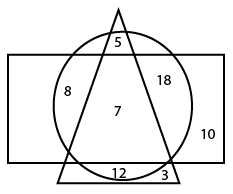Question 10

# In the following diagram, the rectangle represents doctors, the triangle represents players and the circle represents philosophers.The numbers in different segments show the number of persons.According to the given diagram, How many players are philosophers but not Doctors?

Solution

number of players who are philosophers$$=5+7+12=24.$$

But 7 doctors are there.

So, number of players are philosophers who are not doctor is $$=24-7=17.$$

Option A is correct.

• Free SSC Study Material - 18000 Questions
• 230+ SSC previous papers with solutions PDF